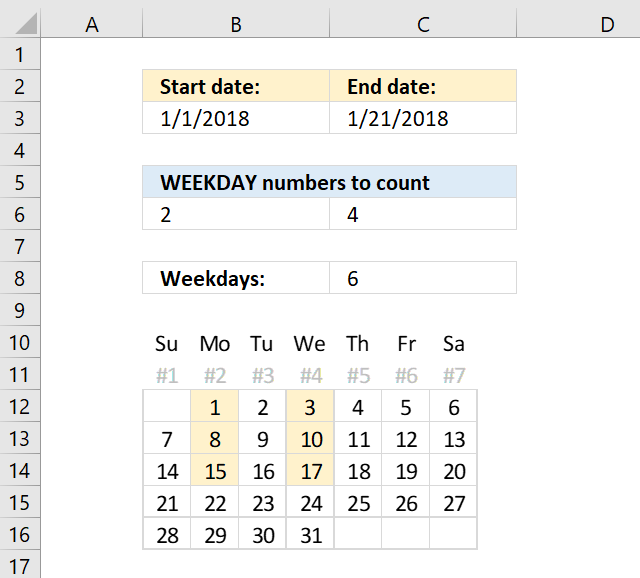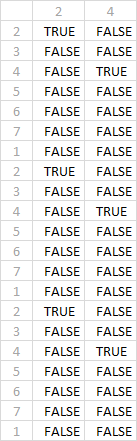Author: Oscar Cronquist Article last updated on March 11, 2019If you want to count specific weekdays like for example Mondays and Wednesdays you need a more complicated array formula.

Formula in cell C8:

=SUMPRODUCT((WEEKDAY(B3+ROW(A1:INDEX(A1:A10000,C3-B3+1))-1)=B6:C6)*1)

### Explaining formula in cell C8

The INDEX function allows you to create a cell range with a specific size. We want it to be the same size as there are days between the start date and end date + 1.

A1:INDEX(A1:A10000,C3-B3+1))

becomes

A1:INDEX(A1:A10000,43121-43101+1))

becomes

A1:INDEX(A1:A10000,21)) and returns A1:A21.

The row function then returns the row number for each cell.

ROW(A1:INDEX(A1:A10000,C3-B3+1))

becomes

ROW(A1:A21) and returns this array {1; 2; 3; ... ; 21}

The array must start with 0 (zero) so we subtract the array with 1.

WEEKDAY(B3+ROW(A1:INDEX(A1:A10000,C3-B3+1))-1

becomes

{1; 2; 3; ... ; 21} - 1 and returns {0; 1; 2; ... ; 20}

It is now time to add the start date to the array.

B3+ROW(A1:INDEX(A1:A10000,C3-B3+1

becomes

43101+{0; 1; 2; ... ; 20} and returns {43101; 43102; ... ;43121}

The WEEKDAYS function converts the dates to numbers of the week. Sunday is 1 and Saturday is 7.

WEEKDAY(B3+ROW(A1:INDEX(A1:A10000,C3-B3+1))-1)

becomes

WEEKDAY({43101; 43102; ... ;43121}) and returns {2; 3; ... ; 1}

We need to compare the values in the array with the weekday numbers we want to count. To count Mondays and Wednesdays we need number 2 and 4.

WEEKDAY(B3+ROW(A1:INDEX(A1:A10000,C3-B3+1))-1)=B6:C6

becomes

{2; 3; 4; 5; 6; 7; 1; 2; 3; 4; 5; 6; 7; 1; 2; 3; 4; 5; 6; 7; 1}={2,4} and returns the following array containing boolean values:The SUMPRODUCT function then sums the numbers but first we need to convert the boolean values to numbers. It is easy, simply multiply with 1.

SUMPRODUCT((WEEKDAY(B3+ROW(A1:INDEX(A1:A10000,C3-B3+1))-1)=B6:C6)*1)

becomes

SUMPRODUCT({1,0; 0,0; 0,1; 0,0; 0,0; 0,0; 0,0; 1,0; 0,0; 0,1; 0,0; 0,0; 0,0; 0,0; 1,0; 0,0; 0,1; 0,0; 0,0; 0,0; 0,0}) and returns 6 in cell C8.